## Product Management / Product Ownership Training

Entdecke die Welt des effektiven Product Managements. Nächster Trainingstermin: 11.-14.12. (Remote) – jetzt buchen!
Zum TrainingArtificial Intelligence

# Deep CSG: Extraction of Geometric Objects from 3D Point Clouds Using Deep Learning

Autor:in
Lesezeit
22 ​​min

Recent research in the fields of Computer Vision and Computer Graphics often addresses the extraction of geometric shapes from 3D point clouds. The processing of raw point clouds is of particular use, because in practice, real objects, e.g. components of a car, are captured using a 3D scanner to subsequently generate a digital 3D model.

Designers then can edit these components in Computer-Aided Design (CAD) programs. Another use case for transferring a 3D point cloud into a 3D model is the automatic construction of 3D building plans based on sensor data. This is also applicable in the robotics domain. A robot, which is supposed to perform certain actions with one or more objects, requires information about their position and shape as well as their connectedness.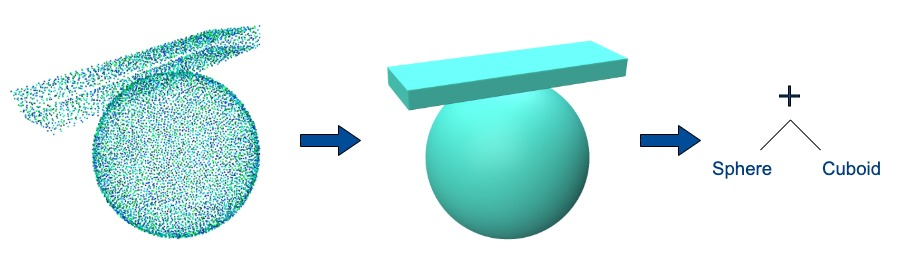The goal is to fit geometric objects to a 3D point cloud to arrange them in a Constructive Solid Geometry (CSG) tree.

## Background

### Constructive Solid Geometry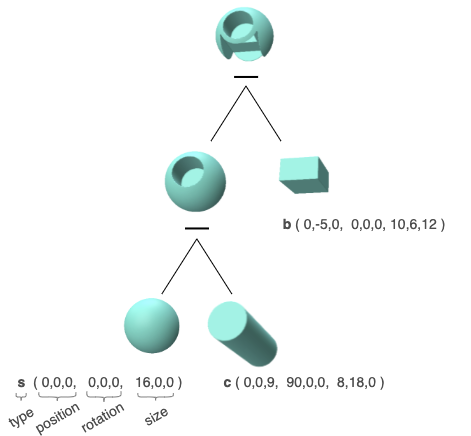Each geometric primitive in the CSG tree is associated with a shape type and a 9-dimensional parameter vector specifying its position, rotation, and size.

One widespread modeling scheme of such 3D models is Constructive Solid Modeling (CSG). It yields a compact and easy-to-edit representation of geometric solids while allowing for the creation of complex appearing objects. The syntax obeys a tree topology whose inner nodes contain elementary Boolean operations and the leaves are geometric primitives. A geometric primitive is a basic object (e.g. a line, a surface or a volumetric shape) whose position, rotation and size can be described by a small set of parameters. Exemplary volumetric primitives are spheres, cylinders, and cuboids. The decomposition of complex objects into such higher-level shapes simplifies their editing and interaction. Unlike low-level shape representations, such as voxels or points, their parameterization provides structural information which is close to human perception and, therefore, is of high value for designers.

### Signed Distance Fields

Another important concept are Signed Distance Fields (SDF). A distance field is a spatial, n-dimensional field that associates a scalar value to each point it encompasses.  This value is the closest distance of the point to a shape within its domain. In an SDF the distances are signed to indicate whether a point lies inside or outside the object’s boundary. Points that are located outside receive a positive distance, those lying on the surface are zero-valued and negative distances mark the interior of a shape.Rendering process from a geometric object (a) to an SDF grid (c) using a discretized 3D bounding volume (b). [Image Source: Miroslava Slavcheva. Signed Distance Fields for Rigid and Deformable 3DReconstruction.]The Signed Distance Function is an n-dimensional function $$\phi: \Omega \subseteq \mathbb{R}^n \rightarrow \mathbb{R}$$ which maps the minimum distance to a specific object to its input. Spheres, cuboids, and cylinders have a unique, differentiable signed distance function each, e.g.  $$\phi_{sphere}(p) = d(p, c) – r$$, where $$d$$ is the distance metric of the underlying metric space (here: Euclidean distance), $$c$$ is the sphere’s center and $$r$$ its radius. Boolean operations also have unique signed distance functions ($$\cup$$: union, $$–$$: difference):

\begin{equation} \begin{aligned}
\phi_{\,\cup\,}(\Phi_1, \Phi_2) &= min(\Phi_1, \Phi_2), \\[1ex]
\phi_{\,-\,}(\Phi_1, \Phi_2) &= max(\Phi_1, \,\phi_{\,\setminus\,}(\Phi_2)), \\[1ex]
\end{aligned}
\end{equation}

where the complement is given by $$\phi_{\,\setminus\,}(\Phi) = – \, \Phi_1$$ and $$\Phi_1$$ and $$\Phi_2$$ denote two SDF representations. In general, such simple equations allow the straightforward generation of an SDF from a CSG tree. Note, that min and max are automatically differentiable in my implementation by PyTorch’s autograd and a suitable gradient shaping.

### Surface Normals

An important characteristic of an SDF is that its gradient approximates the normals of the acquired object as it points towards the direction of the steepest (positive or negative) slope. Surface normals point towards a direction perpendicular to an object’s surface and define the orientation of the shape. They are used, for instance, in the field of Computer Graphics to apply visual effects as shading.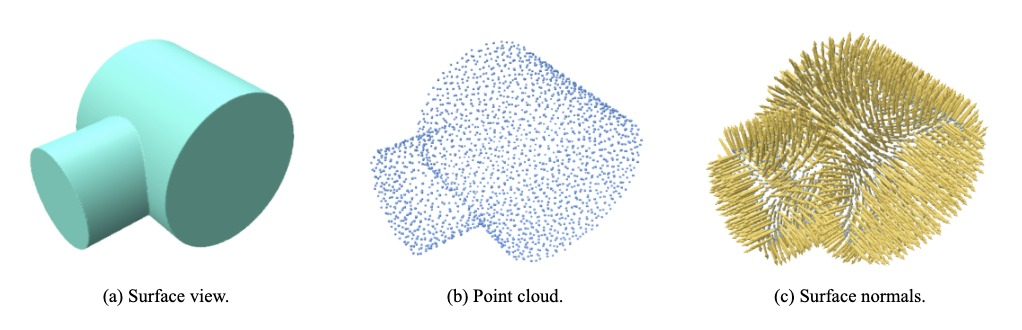a) Surface view of a geometric object. b) 3D point cloud of the geometric object, comprising only surface points. c) Point cloud with displayed normal vectors, one for each surface point.

## Related work

These are the main related works:

• CSGNet by Sharma et al.: Sharma et al. apply a supervised learning approach to convert 3D voxel grids to CSG trees. Subsequently, a variety of optimization and fine tuning techniques are applied to improve the pre-trained model. The major drawbacks are: (1) The rendering engine is not differentiable, i.e. no gradient-based methods are applicable to incorporate visual feedback into the optimization process and (2) In practice, objects are captured as point clouds by 3D scanners, i.e. voxel grids simplify the problem.
• SPFN by Li et al.: The goal is to fit a set of primitives to a 3D point cloud. PointNet++ by Qi et al. is applied as a point cloud encoder. The framework produces good results, but the detected shapes are not expressed as CSG trees. Moreover, they are not as complex as a CSG tree could describe.
• UCSG-NET by Kacper et al.: The framework generates CSG trees to approximate 3D point clouds. Primitives are predicted in the first step and subsequently concatenated by a sequence of network layers. In this work, CSG trees are generated, but a limited set of primitive types is used (only cuboids).

## Concept of Deep CSG

To overcome the drawbacks of the related works introduced previously, a deep learning pipeline, called Deep CSG, was developed as follows: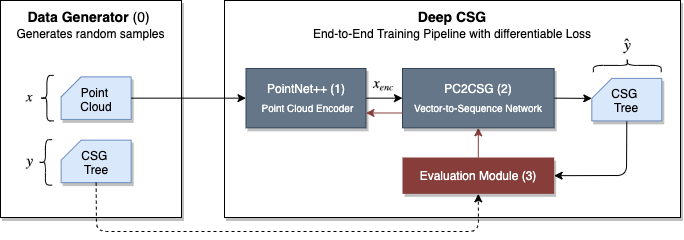Deep CSG pipeline consisting of a data generator (0), a point cloud encoder (1), a CSG predictor (2), and an evaluation module (3).

The first module in the pipeline is a data generator. It generates an arbitrary number of 3D point clouds for training paired with CSG trees for evaluation. Allowed primitives are spheres, cuboids, and cylinders. They can be concatenated by the difference and union operator.

To infer structural, geometric information from point clouds, these have to be preprocessed by a robust point cloud encoder. Point clouds are unordered sets of points and, therefore, a permutation-invariant computation of features is a central cornerstone in their processing. A commonly used point cloud encoder, which satisfies this criterion, is PointNet or its successor PointNet++. Here, the latter is used.

The encoded point clouds are transferred to the PC2CSG network, a vector-to-sequence network, which transforms them into a sequential CSG expression. It employs an RNN  which helps to solve two kinds of problems inherent in CSG sequence prediction: First, the varying size of the trees, and second, the significance of the instruction arrangement.

The figure shows a CSG tree and the corresponding CSG sequence padded to a length of 5. Each instruction $$i$$ in the CSG sequence contains a geometric primitive and an operation. The primitive is expressed by its type $$\in \{s, c, b, \bullet \}$$ and a 9-dimensional parameter vector specifying its position. rotation, and size. The operation is given by its type $$\in \{+, -, \circ \}$$. $$\circ$$ is the unary identity operation, $$\bullet$$ is an empty primitive. Both are neutral classes which do not change the geometry. They are used to pad all CSG sequences to the same length.

The CSG sequences are then evaluated in the evaluation module. Backpropagation is applied to optimize all pipeline components in an end-to-end manner.

## Loss functions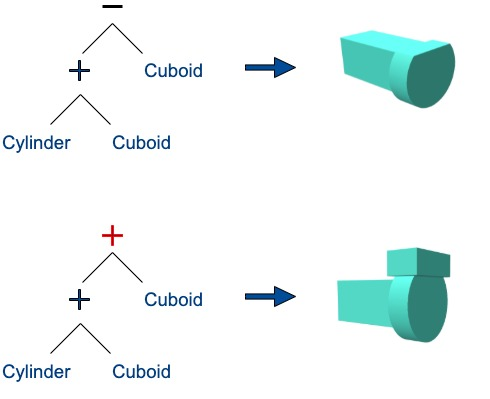The depicted CSG trees differ in one CSG instruction. Despite the nearly identical CSG syntax, the result is a significantly different geometry.

Main focus in this work is on measuring visual aspects. A loss that relies on visual feedback results in a more robust training than a simple comparison of CSG trees. The reason for this is that a slight change in the CSG tree can cause a major change in the rendered geometry. Thus, when applying supervised learning directly to CSG expressions, minor deviations can mislead the algorithm and, consequently, degrade performance. Another weakness of expression-based learning is that, in practice, an infinite number of paths exist to generate a shape. However, during training, each model output is matched against exactly one given target sequence. As a result, a similar geometry, which is underlying a different CSG syntax, is penalized.

To optimize the pipeline components, a variety of loss functions were implemented and investigated in detail. In the following, these loss functions are presented briefly.

### SDF loss

In all experiments, the primary loss function is the SDF distance, which captures grid-based, visual discrepancies between two geometries. It is given by

\begin{equation}\label{equ:sdf-loss}
\begin{aligned}
\mathcal{L}_{SDF} = \frac{1}{|\mathcal{G}|} \; \sum_{x \,\in\, \mathcal{G}} \: 1 – e^{-(\:\phi_y(x) – \:\phi_{\hat{y}}(x))^2}
\end{aligned}
\end{equation}

where y and $$\hat{y}$$ are the target and predicted CSG expressions, $$\mathcal{G}$$ is a regular grid used to discretize the SDF distances and $$\phi$$ is the respective signed distance function. The MSE of the signed distances is scaled to ]0,1] using the exponential function. MSE was preferred over MAE and Huber loss to strongly penalize geometric deviations.Exemplary SDF representations of two 2D objects, which were discretized on a 2D boundary volume to be compared.

### Sequence loss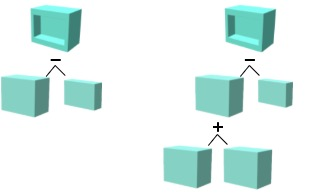The sequence loss regulates the predicted sequence lengths. Since shorter expressions are preferred because, among other reasons, the probability of redundant instructions is inferior, a penalty term is computed only for those that exceed the given ground truth length.  The neutral operations ￮  implicitly indicate the sequence length. Based on them, a binary classification task can be inferred, which can be solved with NLLL.

The figure on the right shows to CSG trees that generate equal geometries. The right CSG tree includes a redundant operation, which is intended to be avoided by the sequence loss.

### Expression loss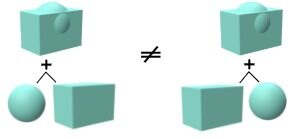To make the meaning of the individual classes more tangible for the algorithm, an expression-based loss for the primitive and operation labels is implemented. NLLL is also used here.

The figure on the right illustrates a disadvantage of the expression loss: Due to the commutative property of the union operator the CSG trees generate equal geometries. An expression-based loss does not take this into account and penalizes the different arrangement of instructions. Owing to the task-inherent shortcomings of supervised learning on labels, the loss is employed merely as a secondary criterion in combination with the SDF loss.

### Parameter loss

The parameter loss serves the purpose of guiding the algorithm to properly interpret the position, rotation, and size of geometric primitives. The parameters are predicted as  regression values and Huber loss is applied. Furthermore, negative sizes and rotations are penalized:

\begin{equation}
\begin{aligned}
\mathcal{L}_{param} =& \sum_{t=1}^T \mathcal{L}_{Huber}(\hat{y}_{t, param},\; y_{t, param}) \, + \, \mathcal{L}_{size} + \mathcal{L}_{rot}, \quad \text{with}
\end{aligned}
\end{equation}

\begin{equation*}
\begin{aligned}
\mathcal{L}_{size} =& \;max(-\hat{y}_{t, size}, 0), \\
\mathcal{L}_{rot} =& \;max(-\hat{y}_{t, rot}, 0).
\end{aligned}
\end{equation*}

$$y_{t, param}$$ is the 9-dimensional parameter vector of the $$t^{th}$$ primitive in the CSG sequence y. $$T$$ is the user-specified maximum number of instructions in a CSG expression (here: 4).

### Sinkhorn loss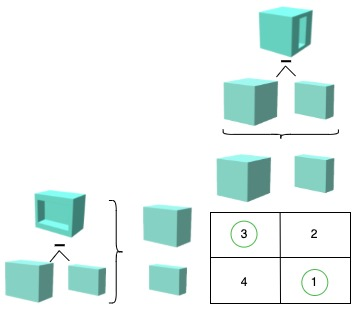The Sinkhorn loss measures the minimal pairwise distance of the individual geometric primitives according to their SDF representation. The differentiable Sinkhorn algorithm searches for the optimal mapping, i.e. the one with the minimum transformation cost. Thus, the constituent geometric primitives are assessed without considering their order and concatenation in the CSG tree. The required cost matrix consists of the pairwise SDF distances between target and predicted primitives. The figure on the right shows a simplified cost matrix containing pairwise SDF distances for two CSG trees. Equal primitives would have 0 costs.

One drawback of the Sinkhorn loss is that, due to the computation of pairwise costs, it is highly computation and memory intensive. Analogous to the Expression loss, the cost is not oriented to the outcome but its constituents.

### Normals loss

Beyond the SDF, the surface normals provide further visual resonance. Learning with this information has the potential to guide a more precise fitting of geometries since the normals define the orientation of a solid. The normal vectors are obtained by applying Finite Differences on the target and predicted CSG tree. The deviation of ground truth and output normals is measured with the cosine distance:

\begin{equation}\label{equ:norm-loss}
\mathcal{L}_{norm} = \frac{1}{P\,} \;(1 – \sum_{p \, \in \, \mathcal{P}} \; cos(\vec{n}_{p}, \hat{\vec{n}}_{p})),
\end{equation}

where P is a set of points located on the target object’s surface and $$\vec{n}_{p} , \hat{\vec{n}}_{p}$$ are the ground truth and predicted surface normals originating from point p.

### Null Object loss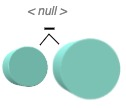The null object loss prevents the model from producing empty objects. These arise when, for example, a larger object is subtracted from a smaller one as shown in the figure on the right or when zero-sized primitives are predicted. To circumvent this, a penalty is imposed if the mean of the K minimum distances in the SDF grid of an object is larger than a given threshold $$\epsilon$$. Additionally, each primitive in the CSG prediction is separately transformed into an SDF and assessed with the Null Object loss to penalize zero-sized primitives. The $$\epsilon$$-threshold is set to $$c – \frac{c}{4}$$, where $$c$$ is the size of a grid cell in the SDF grid. It was empirically established that a relaxed threshold of $$c – \frac{c}{4}$$ is more robust than a strict threshold of $$c$$ since then zero-sized primitives are consistently penalized, while primitives with the smallest size allowed achieve zero loss. In this analysis, $$K = 16$$ was empirically found to be an appropriate choice.

### Diversity loss

The goal of the diversity loss is to restrict the network from favoring one primitive, parameter, or operation class over the others in the output CSG trees. This is accomplished by maximizing the entropy of the available annotations. To turn this into a minimization problem, first, the entropy H is projected onto the interval [0,1] by dividing it by $$log(Z)$$,  where Z is the number of events in $$\mathcal{Z}$$. This yields $$H^{[0,1]}$$:

\begin{equation}
H^{[0,1]}(x) = – \frac{1}{log(Z)} \; \sum_{z\: \in\: \mathcal{Z}} P(z) \; log(P(z)).
\end{equation}

Finally, $$1 – H^{[0,1]}$$ is minimized.

## Evaluation

In each experiment, the primary loss is the SDF loss. All remaining losses presented are used as secondary losses only. To determine the contribution of each secondary loss an ablation study was performed. For each experiment, the optimized loss $$\mathcal{L}^*$$  is given by: $$\mathcal{L}^*= \mathcal{L}_{SDF} + \mathcal{L}_{sec}$$, where $$\mathcal{L}_{sec}$$ is the respective secondary loss. One constraint-based losses, which was tested was the Modified Differential Method of Mutlipliers, but it did not yield good results.

### Evaluation Criteria

The most informative evaluation criteria were the SDF loss, the null object loss, the entropy of CSG sequence length (the higher the better) and the self-developed shape composition score (the lower the better). To obtain the shape composition score, first, a histogram is generated for the target and predicted CSG tree counting the occurrences of spheres, cuboids, and cylinders. Subsequently, pairwise absolute differences between the histograms are calculated. The idea is that, e.g. a spherical surface can be ideally approximated by a sphere and rather insufficiently by a cuboid or a cylinder. Consequently, if the target CSG tree contains a sphere, then, the surface of the described geometry is spherical somewhere. Thus, the output is expected to include a sphere, too.

### Ablation Study

During the ablation study, some models adopted an undesired behavior to gain comparatively good SDF scores in the short term. Without an appropriate penalization, some models produce almost only CSG structures consisting of singular primitives. This was true for the experiments examining Sinkhorn, null object, and normals loss. Conversely, other models like the ones with enabled expression, sequence and parameter loss, which were given a direct or indirect sequence length constraint, attempted to match the first primitive as accurately as possible to the point cloud and then artificially padded the sequence with zero-sized primitives. Additionally, the model with parameter loss performed comparatively well in terms of the SDF loss, although it assigns the same rotation and position of $$(0,0,0)$$ for each primitive.

In general, the ablation study did not succeed in training a model that can reliably build CSG trees. Rather, the prediction of undesirable CSG structures induces a premature convergence to results which are not optimal. On the one hand, this reveals a weakness of the SDF loss which initially can be lowered rapidly by placing singular primitives in the center of the canvas. On the other hand, this shows that one secondary criterion is not sufficient to ensure the desired characteristics of a CSG structure, since the models will find shortcuts to not be penalized. However, the most promising losses are SDF, expression, parameter, and null object loss.

### Final Run

Based on the findings of the ablation study, the final loss was defined as follows $$\mathcal{L}^*= \mathcal{L}_{SDF} + \mathcal{L}_{expr} + \mathcal{L}_{param} + \mathcal{L}_{nullobj}$$.

### Evaluation Criteria

The following table shows the evaluation results of the best experiments of the ablation study, as well as the final run. The best results are in bold:

 Entropy of seq. lengths Null object loss  (%) Shape composition score SDF loss Cuboid Cylinder Sphere $$\mathcal{L}_{SDF}$$ (baseline) 0.0469 0.92 0.6902 0.9144 0.7223 0.8923 $$\mathcal{L}_{SDF} + \mathcal{L}_{expr}$$ 0.8390 17.45 0.4430 0.4780 0.4073 0.8838 $$\mathcal{L}_{SDF} + \mathcal{L}_{param}$$ 0.6452 12.26 0.8570 1.0015 0.7803 0.8619 $$\mathcal{L}_{SDF} + \mathcal{L}_{nullobj}$$ 0.0399 0.24 0.7036 0.9166 0.7193 0.8773 $$\mathcal{L}^*$$ (final) 0.8615 1.26 0.3728 0.3910 0.3623 0.7929

The dashed, green line in the following figure shows the SDF loss of the final experiment at the timestamp of evaluation. Training continued for approximately one month after evaluation and the model continued to improve steadily. This demonstrates that the training process is very slow and tedious.

#### Visual Investigation

In addition, a visual investigation was conducted (see figure below). The top row shows the ground truth, the second the predictions, and the bottom row shows the respective SDF losses. We can see that the predicted CSG expressions are close to the ground truth and the SDF loss of the first five samples is very low. However, the last one is very high. This illustrates the strong penalization of geometric deviations.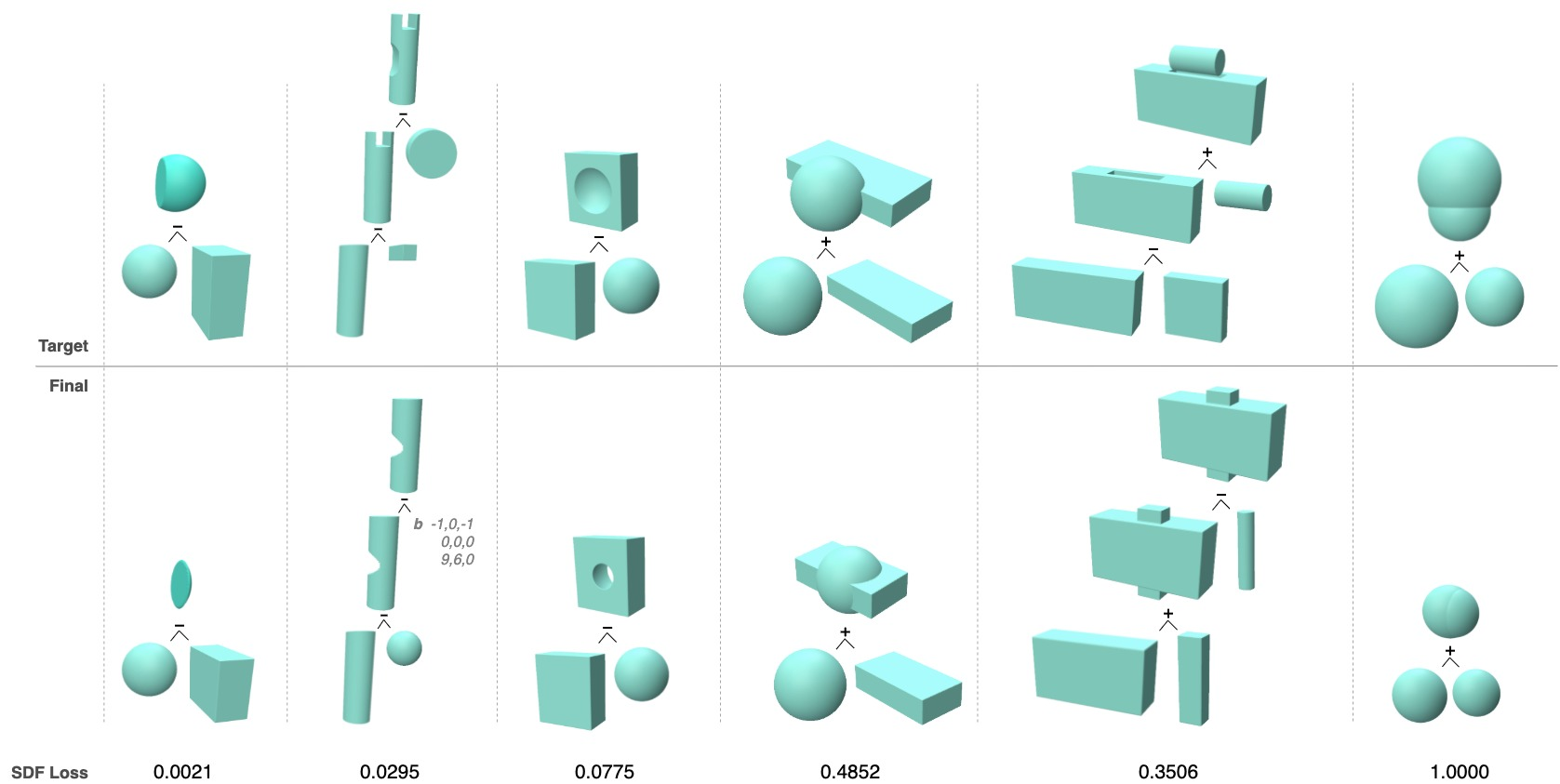The final experiment discards some unwanted patterns of behavior through the combination of losses. Simultaneously activating the expression and null object loss causes the model to fit its outputs to the given sequence lengths while avoiding artificially padding them with zero-sized primitives.  Concurrently, the parameter loss guides the algorithm to minimize the reconstruction error of primitive parameters. The visual investigation and the shape composition score show that the algorithm purposefully assigns primitive and operation types to unseen samples. All these factors provide a significant performance gain in terms of SDF loss.

Similar to the separate experiment on parameter loss, the predictions include two distinct position values, whereas the output space of the size parameters is highly diverse.  Since the first primitive of each ground truth CSG expression is centered, the remaining primitives are far more likely to reside near the center than at the edge of the canvas. To stabilize the performance, it is conceivable that the algorithm commits to a small number of positions near the center at an early stage of training. Constantly predicting positions close to the center yields a comparatively small parameter loss and allows the model to focus on optimizing the diverse primitive sizes. In a proceeding work, the model training could be continued with an additional diversity loss dealing with regression values to diversify the value range of the position parameters.

## Conclusion

In a future work, the rotations could be specified in the form of Quaternions. In the research fields of Computer Vision and Computer Graphics, they are widely used to compute 3D rotations, due to their simplicity and mathematical sophistication. One example, where quaternions are applied, is the UCSG-NET framework. A further improvement could be achieved by integrating Beam Search as Sharma et. al have done with the CSGNet model. This enabled the authors to gain a significant performance increase. Finally, the underlying problem could be simplified by operating on regular data representations, such as voxel grids as proposed by the CSGNet authors, instead of unordered point clouds. As a result, PointNet++ could be substituted with a convolutional neural network which serves as a voxel encoder. However, this modification causes the application to be less practically oriented.